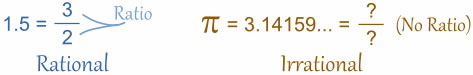# Difference between Irrational and Rational Numbers

##### Key Difference: Rational number is a number that can be expressed in the form of a fraction but with a non-zero denominator. An irrational number is just opposite of a rational number, as it cannot be expressed in the form of a fraction with a non-zero denominator.A number is referred to as a mathematical value that is represented through a word, symbol or figure. Numbers are used to define a particular quantity. Numbers are generally used for measuring, labeling and ordering. Numbers are categorized according to their types. One such category is based on Rational and Irrational Numbers. Rational number is a number that can be expressed in the form of a fraction but with a non-zero denominator. In other words, a rational number can be expressed as the quotient of two integers (with a non-zero denominator). All repeating decimals fall in the category of rational numbers. One can place any rational number exactly on the number line.

Irrational numbers are just opposites of Rational numbers, as they cannot be expressed in the form of fractions with non-zero denominators. In other words, Irrational numbers can be expressed as the quotient of two integers. It is important to mention that many square roots, cube roots, etc. fall in the category of irrational numbers. However, all roots are not irrational numbers. Irrational numbers can be expressed as non-terminating, non-repeating decimals.

Comparison between Irrational and Rational Numbers:

 Rational Numbers Irrational Numbers Definition Rational number is a number that can be expressed in the form of a fraction but with a non-zero denominator. Irrational numbers are just opposites of Rational numbers, as they cannot be expressed in the form of a fraction with a non-zero denominator. Examples 8, as 8 can be expressed in the form of (8/1) 3/4 , as it is in a fraction form 0/3, as it in a fraction form Square root of 16, as it would be 4 and that can be expressed as (4/1) .7777777, all repeating decimals are rational. .12, as it can be expressed as 12/10 Square root of five, as it cannot be simplified further. 4/0, as the denominator is not non zero Pi (3.14159265358….) is the most well known irrational number. However, in certain calculations the approximate value of pi is considered Euler’s number (2.7182818284……) Golden Ratio (1.6180339887………) Resulted from Actual measurement Theoretical  calculation or a definition

Image Courtesy: mathsisfun.com

## Comments

Thanks for the help.. After reading this, I really understand what rational and irrational numbers are :)

## Add new comment

### Plain text

CAPTCHA
This question is for testing whether or not you are a human visitor and to prevent automated spam submissions.